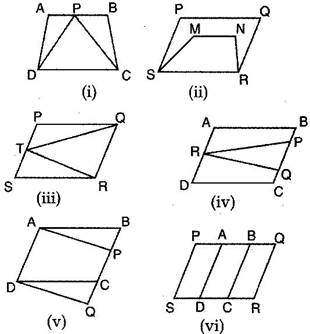### Areas of Parallelograms and Triangles - Solutions

CBSE Class 9 Mathematics

NCERT Solutions
CHAPTER 9
Areas of Parallelograms and Triangles(Ex. 9.1)

1. Which of the following figures lie on the same base and between the same parallels. In such a case, write the common base and the two parallels.Ans.
In figure (i): Triangle DPC and trap. ABCD are on the same base DC and between same parallel DC and AB.

In figure (iii): triangle RTQ and parallelogram PQRS are on the same base QR and between same parallel QR and PS.

In figure (v): Parallelogram ABCD and parallelogram APQD are on the same base AD and between the same parallels AD and BQ.